# Pulley system on a double inclined plane

(diff) ← Older revision | Latest revision (diff) | Newer revision → (diff)
This article is about the following scenario. A fixed triangular wedge has two inclines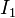$I_1$ and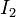$I_2$ making angles$\alpha_1$ and$\alpha_2$ with the horizontal. A pulley is affixed to the top vertex of the triangle. A string through the pulley has attached at its two ends blocks of masses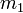$m_1$ and$m_2$, resting on the two inclines$I_1$ and$I_2$ respectively. The string is inextensible. The coefficients of static and kinetic friction between$m_1$ and$I_1$ are$\mu_{s1}$ and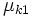$\mu_{k1}$ respectively. The coefficients of static and kinetic friction between$m_2$ and$I_2$ are$\mu_{s2}$ and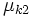$\mu_{k2}$ respectively. Assume that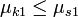$\mu_{k1} \le \mu_{s1}$ and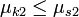$\mu_{k2} \le \mu_{s2}$.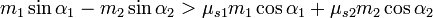$\! m_1\sin \alpha_1 - m_2\sin \alpha_2 > \mu_{s1}m_1\cos \alpha_1 + \mu_{s2}m_2\cos \alpha_2$$m_1$ slides downward and$m_2$ slides upward, with the same magnitude of acceleration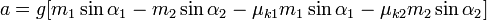$\! a = g[m_1 \sin \alpha_1 - m_2 \sin \alpha_2 - \mu_{k1}m_1\sin \alpha_1 - \mu_{k2}m_2 \sin\alpha_2]$.$\! m_2\sin \alpha_2 - m_1\sin \alpha_1 > \mu_{s1}m_1\cos \alpha_1 + \mu_{s2}m_2\cos \alpha_2$$m_2$ slides downward and$m_1$ slides upward, with the same magnitude of acceleration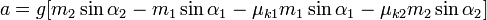$\! a = g[m_2 \sin \alpha_2 - m_1 \sin \alpha_1 - \mu_{k1}m_1\sin \alpha_1 - \mu_{k2}m_2 \sin\alpha_2]$.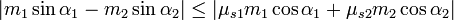$\! |m_1 \sin \alpha_1 - m_2 \sin \alpha_2| \le |\mu_{s1}m_1 \cos \alpha_1 + \mu_{s2}m_2 \cos \alpha_2|$ The system remains at rest$0$